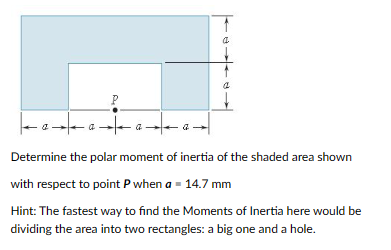# (Solved): Determine the polar moment of inertia of the shaded area shown with respect to point P when a=14.7 ...Determine the polar moment of inertia of the shaded area shown with respect to point when Hint: The fastest way to find the Moments of Inertia here would be dividing the area into two rectangles: a big one and a hole.

We have an Answer from Expert

Attached image shows the X-X axis and Y-Y axis.

Moment of inertia of shaded area about the X-X axis (shown in the attached image):-

Axis X-X is the base of the rectangle section.
Therefore,

We have an Answer from Expert